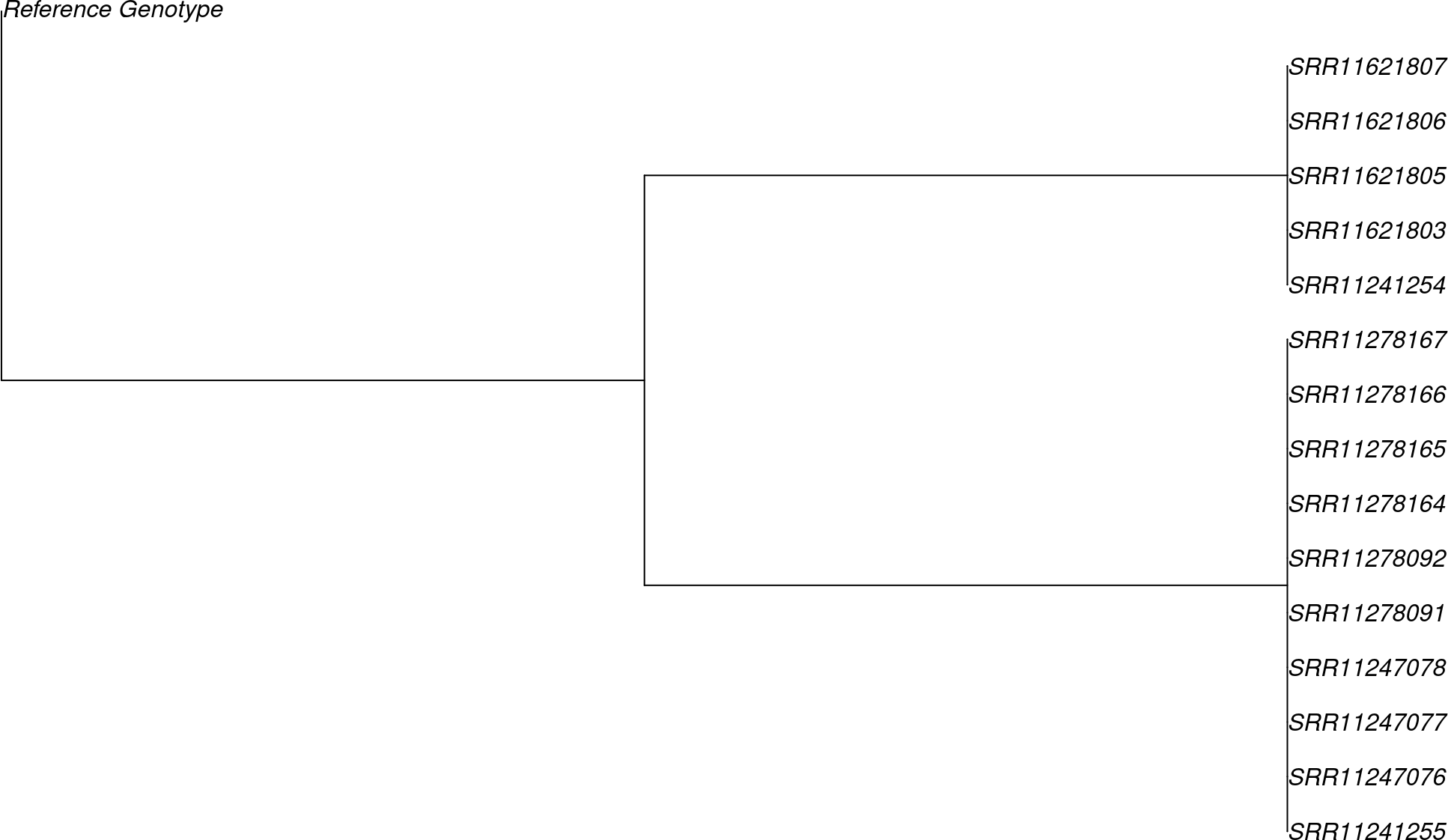## 0.1 Example of phylogenetic inference by VERSO

We now present an example of phylogenetic analysis by VERSO using mutation data from a set of SARS-CoV-2 samples; the dataset includes variants for a selected set of 15 SARS-CoV-2 samples obtained by variant calling from raw data available from NCBI BioProject PRJNA610428.

We first load the data. Notice that the input data to VERSO is an array reporting variants either observed (as 1 in the matrix), not observed (as 0) or missing (as NA, i.e., due to low coverage).

library("VERSO")
data(variants)

We setup the main parameter in oder to perform the inference. The first main parameter to be defined as input is represented by the false positive and false negative error rates, i.e., alpha and beta. When multiple set of rates are provided, VERSO performs a grid search in order to estimate the best set of error rates.

alpha = c(0.01,0.05)
beta = c(0.01,0.05)
head(alpha)
##  0.01 0.05
head(beta)
##  0.01 0.05

We can now perform the inference as follow. Make sure to set the random seed to ensure reproducibility.

set.seed(12345)
inference = VERSO(D = variants,
alpha = alpha,
beta = beta,
check_indistinguishable = TRUE,
num_rs = 5,
num_iter = 100,
n_try_bs = 50,
num_processes = 1,
verbose = FALSE)

We notice that the inference resulting on the command above should be considered only as an example; the parameters num rs, num iter and n try bs representing the number of steps perfomed during the inference are downscaled to reduce execution time. We refer to the Manual for discussion on default values. We provide within the package results of the inference performed with the same parameters as RData.

data(inference)
print(names(inference))
##  "B"                    "C"                    "phylogenetic_tree"
##  "corrected_genotypes"  "genotypes_prevalence" "genotypes_summary"
##  "log_likelihood"       "error_rates"

VERSO returns a list of 8 elements as results. Namely, B, C, phylogenetic tree, corrected genotypes, genotypes prevalence, genotypes summary, log likelihood and error rates. Here, B returns the maximum likelihood variants tree (inner nodes of the phylogenetic tree), C the attachment of patients to genotypes and phylogenetic tree VERSO phylogenetic tree, including both variants tree and patients attachments to variants; corrected genotypes is the corrected genotypes, which corrects D given VERSO phylogenetic tree, genotypes prevalence the number of patients and observed prevalence of each genotype and genotypes summary provide a summary of association of mutations to genotypes; finally log likelihood and error rates return the likelihood of the inferred phylogenetic moldel and best values of alpha and beta as estimated by VERSO.

We can plot the inferred phylogetic tree using the function plot from the package ape.

plot(inference\$phylogenetic_tree)